# DAV Class 7 Maths Chapter 7 Worksheet 1 Solutions

The DAV Maths Book Class 7 Solutions Pdf and DAV Class 7 Maths Chapter 7 Worksheet 1 Solutions of Linear Equations in One Variable offer comprehensive answers to textbook questions.

## DAV Class 7 Maths Ch 7 WS 1 Solutions

Question 1.
(i) 5x – 2 = 18
5x – 2 = 18
⇒ 5x – 2 + 2 = 18 + 2
⇒ 5x = 20
⇒ 5x + 5 =20 + 5
⇒ x = 4
Check: L.H.S. = 5 × 4 – 2 = 20 – 2 = 18
R.H.S. = 18
Hence, x = 4 is the solution of the given equation.

(ii) $$\frac{1}{4}$$y + $$\frac{1}{2}$$ = 5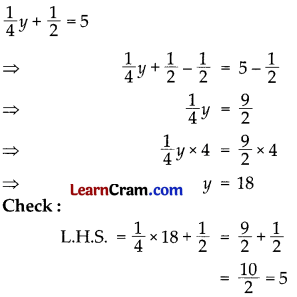(iii) $$\frac{3}{5}$$x – 6 = 3R.H.S = 3
Hence, x = 15 is the solution of the given equation.

(iv) 3x + $$\frac{1}{5}$$ = 2 – xHence, x = $$\frac{9}{20}$$ is the solution of the given equation.

(v) 8x + 5 = 6x – 5
8x + 5 = 6x – 5
⇒ 8x – 6x + 5 = -5
⇒ 2x + 5 = -5
⇒ 2x + 5 – 5 = -5 – 5
⇒ 2x = -10
∴ x = – 5
Check: L.H.S. = 8 × -5 + 5 = -40 + 5
= -35
R.H.S. = 6 × -5 -5 = -30 – 5 = -35
L.H.S. = R.H.S.
Hence, x = – 5 is the solution of the given equation.

(vi) 9z – 13 = 11z + 27
9z – 13 = 11z + 27
⇒ 9z – 11z -13 = 27
⇒ -2z -13 = 27
⇒ – 2z -13 + 13 = 27 + 13
⇒ -2z = 40
⇒ z = – 20
Check: L.H.S. = 9 × – 20 -13 = -180 -13
= -193
R.H.S. = 11 × -20 + 27 – = – 220 + 27
= -193
L.H.S. = R.H.S.
Hence, z = – 20 is the solution of the given equation.

(vii) $$\frac{7}{y}$$ + 1 = 29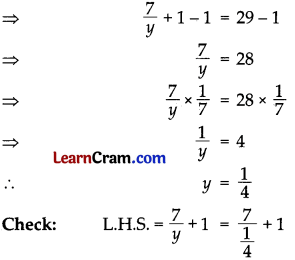= 7 × 4 + 1
= 28 + 1 = 29
R.H.S. = 29
L.H.S. = R.H.S.
Hence, y = $$\frac{1}{4}$$ is the solution of the given equation.(viii) $$\frac{3}{5}$$x + $$\frac{2}{5}$$ = 1R.H.S = 1
L.H.S = R.H.S
Hence, x = 1 is the solution of the given equation.

(ix) 4y – 2 = $$\frac{1}{5}$$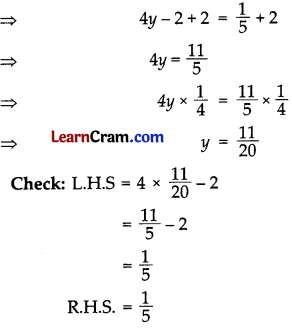Hence, y = $$\frac{11}{5}$$

(x) $$\frac{x}{2}$$ + $$\frac{x}{4}$$ = 12R.H.S = 12
L.H.S = R.H.S
Hence, x = 16 is the solution of the given equation.

(xi) $$\frac{2}{5}$$z = $$\frac{3}{8}$$z + $$\frac{7}{20}$$L.H.S = R.H.S
Hence, z = 14 is the solution of the given equation.

(xii) $$\frac{2}{5}$$y – $$\frac{5}{8}$$y = $$\frac{5}{12}$$L.H.S = R.H.S
Hence, y = $$\frac{-50}{27}$$ is the solution of the given equation.

(xiii) 3x + 2 (x + 2) = 20 – (2x – 5)
3x + 2 (x + 2) = 20 – (2x – 5)
⇒ 3x + 2x + 4 = 20 – 2x + 5
⇒ 5x + 4 = – 2x + 25
⇒ 5x + 2x = 25 – 4
⇒ 7x = 21
x = 3
Check L.H.S = 3 × 3 + 2 (3 + 2)
= 9 + 2 × 5
= 9 + 10 = 19
R.H.S = 20 – (2 × 3 – 5)
= 20 – (6 – 5)
= 20 – 1 = 19
L.H.S. = R.H.S.
Hence, x = 3 is the solution of the given

(xiv) 13(y – 4) – 3 (y – 9) = 5(y + 4)
13(y – 4) – 3 (y – 9) = 5(y + 4)
⇒ 13y – 52 – 3y + 27 = 5y + 20
⇒ 10y – 25 = 5y + 20
⇒ 10y – 5y = 25 + 20
⇒ 5y = 45
⇒ y = 9
Check: L.H.S = 13(9 – 4) – 3 (9 – 9)
= 13 × 5 – 3 × 0
= 65 – 0 = 65
5(9 + 4) = 5 × 13 = 65
L.H.S. = R.H.S.
Hence, y = 9 is the solution of the given equation.(xv) (2z – 7) – 3 (3z + 8) = 4z – 9
(2z – 7) – 3(3z + 8) = 4z – 9
⇒ 2z – 7 – 9z – 24 = 4z – 9
⇒ – 7z – 31 = 4z – 9
⇒ – 7z – 4z = 31 – 9
⇒ – 11z = 22
∴ z = – 2
Check: L.H.S. = (2x – 2 – 7) – 3(3x – 2 + 8)
= (- 4 – 7) – 3 (- 6 + 8)
= -11 – 3(2)
= -11 – 6 = -17
R.H.S. = 4 × -2 – 9 = -8 – 9 = -17
L.H.S. = R.H.S.
Hence, z = – 2 is the solution of the given equation.

(xvi) 4(2y – 3) + 5(3y – 4) = 14
4(2y – 3) + 5 (3y – 4) = 14
⇒ 8y -12 + 15y – 20 = 14
⇒ 23y – 32 = 14
⇒ 23y = 14 + 32
⇒ 23y = 46
∴ y = 46 + 23
y = 2
Check: L.H.S. = 4(2 × 2 – 3) + 5 (3 × 2 – 4)
= 4(4 – 3) + 5 (6 – 4)
=4 × 1 + 5 × 2
= 4 + 10
= 14
R.H.S. = 14
L.H.S. = R.H.S.
Hence, y = 2 is the solution of the given equation.

(xvii) $$\frac{x}{2}-\frac{x}{3}=\frac{x}{4}+\frac{1}{2}$$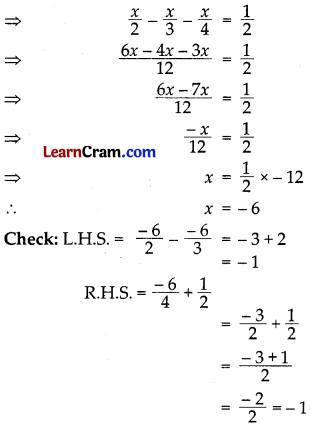L.H.S = R.H.S
Hemce, x = -6 is the solution of the given equation

(xviii) z – $$\frac{x}{2}-\frac{x}{3}=\frac{x}{4}+\frac{1}{2}$$ = 5
⇒ $$\frac{6 z-4 z+3 z}{6}$$ = 5
⇒ 9z – 4z = 5 × 6
⇒ 5z = 30
⇒ z = 30 ÷ 5 = 6
z = 6
Checks: L.H.S = 6 – $$\frac{2 \times 6}{3}+\frac{6}{2}$$ = 6 – 4 +3
= 5 = R.H.S
Hence, z = 6 is the solution of the given equation.

(xix) $$\frac{6 y+1}{2}$$ + 1 = $$\frac{7 y-3}{3}$$
⇒ $$\frac{6 y+1+2}{2}=\frac{7 y-3}{3}$$
⇒ $$\frac{6 y+3}{2}=\frac{7 y-3}{3}$$
⇒ 3(6y + 3) = 2(7y – 3)
⇒ 18y + 9 = 14y – 6
⇒ 18y – 14y = -9 – 6
⇒ 4y = – 15L.H.S = R.H.S
Hence, y = $$\frac{-15}{4}$$ is the solution of the given equation.(xx) $$\frac{6 x-2}{5}=\frac{2 x-1}{3}-\frac{1}{3}$$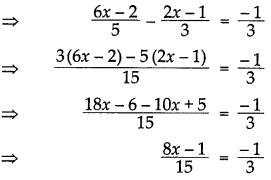⇒ 3(8x – 1) = – 1 × 15
⇒ 24x – 3 = -15
⇒ 24x = -15 + 3
⇒ 24x = -12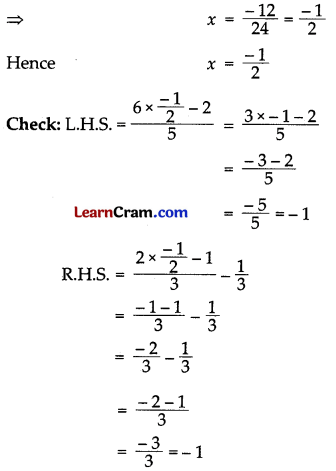L.H.S = R.H.S
Hence, x = –$$\frac{1}{2}$$ is the solution of the given equation.

(xxi) $$\frac{z-1}{3}=1+\frac{z-2}{4}$$
⇒ $$\frac{z-1}{3}=\frac{4+z-2}{4}$$
⇒ $$\frac{z-1}{3}=\frac{z+2}{4}$$
⇒ 4(z – 1) = 3(z + 2)
⇒ 4z – 4 = 3z + 6
⇒ 4z – 3z = 4 + 6
⇒ z = 10

Check: L.H.S = $$\frac{10-1}{3}=\frac{9}{3}$$ = 3
R.H.S = 1 + $$\frac{10-2}{4}$$
= 1 + $$\frac{8}{4}$$
= 1 + 2 = 3
L.H.S = R.H.S
Hence, z = 10 is the solution of the given equation.

(xxii) 2x – 3 = $$\frac{3}{10}$$(5x – 12)
⇒ 10(2x – 3) = 3(5x – 12)
⇒ 20x – 30 = 15x – 36
⇒ 20x – 15x = -36 + 30
⇒ 5x = -6L.H.S = R.H.S
Hence, x = $$\frac{-6}{5}$$ is the solution of the given equation.(xxiii) 3(y – 3) = 5(2y + 1)
⇒ 3y – 9 = 10y + 5
⇒ 3y – 10y = 9 + 5
⇒ – 7y = 14
⇒ y = -2

Check: L.H.S = 3(-2 – 3) = 3 × -5
= -15

R.H.S = 5(2 × -2 + 1) = 5(-4 + 1)
= 5 × – 3
= -15
L.H.S = R.H.S
Hence, y = -2 is the solution of the given equation.

(xxiv) 0.6x + 0.8 = 0.28x + 1.16
⇒ 0.6x – 0.28x = 1.16 – 0.8
⇒ 0.32x = 0.36L.H.S = R.H.S
Hence, x = $$\frac{9}{8}$$ is the solution of the given equation.

(xxv) 2.4(3 – x) – 0.6 (2x – 3) = 0
⇒ 2.4 × 3 – 2.4x – 0.6 × 2x + 0.6 × 3 = 0
⇒ 7.2 – 2.4x – 1.2x +1.8 = 0
⇒ – 3.6x + 9.0 =0
⇒ -3.6x = -9.0
⇒ x = $$\frac{9}{3.6}=\frac{5}{2}$$
= 2.5
Check: L.H.S. = 2.4 (3 – 2.5) – 0.6 (2 × 2.5 – 3)
= 2.4 × 0.5 – 0.6 (5 – 3)
= 1.20 – 0.6 × 2
= 1.20 – 1.20
= 0 = R.H.S.
L.H.S. = R.H.S.
Hence, x = 2.5 is the solution of the given equation.### DAV Class 8 Maths Chapter 7 Worksheet 1 Notes

• Equation is a statement of equality having one or more variables.
e.g. 3x – 5 = 0
x2 + 5x – 3 = 0,
y2 – 4 = 0,
2x + 3y = 7 etc.
• Linear equation in one variable is the equation in which the highest power of the variable is one.
e.g. 3x + 1 = 2x – 3,
$$\frac{5}{6}$$ x – $$\frac{1}{3}$$ = 4x + 5,
2 (x – 3) = 3 (2x + 5)
• The standard form of linear equation in one variable x is ax + b = 0, where a and b are rationals and a ≠ 0.
• The general solution of ax + b = 0 is given by x = – b/a.

Rules:

1. Adding the same quantity to both sides of an equation, makes no difference.
2. Subtracting same quantity from both sides of an equation, makes no difference.
3. Multiplying both sides of an equation by the same non-zero quantity, makes no difference.
4. Dividing both sides of an equation by the same non-zero quantity makes no difference.
5. When a term is transposed from one side to another, its sign is changed.
For example, + changes to – and vice-versa × changes to ÷ and vice-versa.

Finding a solution to a word problem involves following three steps:

• Forming an equation.
• Solving an equation.
• Interpreting the solution.Example 1:
Solve $$\frac{2 x}{3}$$ – 1 = $$\frac{1}{3}$$ and check the answer.
$$\frac{2 x}{3}$$ – 1 = $$\frac{1}{3}$$
$$\frac{2 x}{3}$$ – 1 + 1 = $$\frac{1}{3}$$ + 1 (adding 1 to bothsides)
$$\frac{2 x}{3}$$ = $$\frac{4}{3}$$
$$\frac{2}{3} x \times \frac{3}{2}=\frac{4}{3} \times \frac{3}{2}$$ (Multiplying both sides by $$\frac{3}{2}$$)
x = 2

Check:
L.H.S. = $$\frac{2}{3}$$ × 2 – 1 = $$\frac{4}{3}$$ – 1
= $$\frac{4-3}{3}$$
= $$\frac{1}{3}$$
R.H.S. = $$\frac{1}{3}$$
Hence x = 2 is the solution of the given equation.

Example 2:
Solve: 3$$\frac{3}{4}$$ x = 5x – 2$$\frac{1}{2}$$
3$$\frac{3}{4}$$ x = 5x – 2$$\frac{1}{2}$$
⇒ $$\frac{15}{4}$$x = 5x – $$\frac{5}{2}$$
⇒ $$\frac{15}{4}$$x – 5x = $$\frac{-5}{2}$$
(Collecting the like terms on same side)
⇒ $$\frac{15 x-20 x}{4}$$ = $$\frac{-5}{2}$$
⇒ $$\frac{-5 x}{4}$$ = $$\frac{-5}{2}$$
⇒ $$\frac{-5 x}{4} \times \frac{-4}{5}=\frac{-5}{2} \times \frac{-4}{5}$$
(Multiplying both sides by $$\frac{-4}{5}$$)
⇒ x = 2.Example 3.
Solve: $$\frac{2}{3}$$ (x – 3) = 1 – $$\frac{5}{6}$$ (3x – 4)
$$\frac{2}{3}$$ (x – 3) = 1 – $$\frac{5}{6}$$ (3x – 4)
⇒ $$\frac{2}{3}$$ x – 3 × $$\frac{2}{3}$$ = 1 – $$\frac{5}{6}$$ × 3x – $$\frac{5}{6}$$ × – 4 (Removing the brackets)
⇒ $$\frac{2}{3}$$ x – 2 = 1 – $$\frac{5}{2}$$ x + $$\frac{10}{3}$$
⇒ $$\frac{2}{3}$$ x + $$\frac{5}{2}$$ x = 1 + $$\frac{10}{3}$$ + 2
(Collecting the like terms on same sides)
⇒ $$\frac{4 x+15 x}{6}=\frac{3+10+6}{3}$$
⇒ $$\frac{19 x}{6}=\frac{19}{3}$$
⇒ $$\frac{19}{6} x \times \frac{6}{19}=\frac{19}{3} \times \frac{6}{19}$$
(Multiplying both sides by $$\frac{6}{19}$$)
⇒ x = 2.

Example 4.
On adding 6 to 4 times a number, we get 30. Find the number.
Let the required number be x
∴ 4x + 6 = 30
⇒ 4x + 6 – 6 = 30 – 6
⇒ 4x = 24
⇒ x = 6
Hence the required number is 6.

Example 5.
The sum of three consecutive natural numbers is 120. Find the numbers.
Let the three natural numbers be x, x + 1, x + 2
∴ x + x + 1 + x + 2 = 120
⇒ 3x + 3 = 120
⇒ 3x + 3 – 3 = 120 – 3
⇒ 3x = 117
⇒ 3x ÷ 3 = 117 ÷ 3
⇒ x = 39
Hence the required numbers are 39, 40 and 41.Example 6.
There are 48 students in a class. If number of boys are double to the number of girls, find the number of boys in the class.